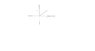# Laplace transform question

math_04

## Homework Statement

This has to do with the unit step function. The question is;

Sketch the function g(t) = 1 for 0<t<1 and is 0 for t>1.

Express g(t) in terms of the unit step function and hence or otherwise show that

L(g(t)) = 1/s^2 - e^-s (1/s^2 + 1/2)

## The Attempt at a Solution

I sketched the graph (see attachment below). I am guessing the unit step function is t(u(t) - u(t-1)).

I tried one way of getting that answer like this t( u(t-1) u(t+1) - u(t-1) ). Obviously it didnt work out. I think I need to get u(t) in the form u(t-a) so I can use the table of Laplace transforms and just read out of it. How do I manipulate this kind of functions?

Thanks.

#### Attachments

•Unit Step function.JPG
2.3 KB · Views: 348

Homework Helper
This is from my notes:

http://img508.imageshack.us/img508/1289/unitstepdk5.jpg [Broken]

In this case, the function should be u(t) - u(t-1).

The Laplace transform you should be using is $$L[f(t-a)u(t-a)] = e^{-as}F(s) \ \mbox{where} \ F(s) = L[f(t)]$$

Note that u(t-a) = f(t-a)u(t-a) where f(t) = 1. f is a constant function.

So you only need to find the laplace transform of 1 and add in the e^(-as) factor in front of it.

Last edited by a moderator: